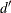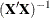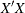The SYSLIN Procedure

MODEL Statement

• MODEL response = regressors / options;

The MODEL statement regresses the response variable on the left side of the equal sign against the regressors listed on the right side.

Models can be given labels. Model labels are used in the printed output to identify the results for different models. Model labels are also used in SRESTRICT and STEST statements to refer to parameters in different models. If no label is specified, the response variable name is used as the label for the model. The model label is specified as follows:

• label : MODEL …;

The following options can be used in the MODEL statement after a slash (/).

ALL

specifies the CORRB, COVB, DW, I, OVERID, PLOT, STB, and XPX options.

ALPHA=value

specifies theparameter for Fuller’s modification to the LIML estimation method. See the section Fuller’s Modification to LIML for details.

CORRB

prints the matrix of estimated correlations between the parameter estimates.

COVB

prints the matrix of estimated covariances between the parameter estimates.

DW

prints Durbin-Watson statistics and autocorrelation coefficients for the residuals. If there are missing values,is calculated according to Savin and White (1978). Use the DW option only if the data set to be analyzed is an ordinary SAS data set with time series observations sorted in time order. The Durbin-Watson test is not valid for models with lagged dependent regressors.

I

prints the inverse of the crossproducts matrix for the model,. If restrictions are specified, the crossproducts matrix printed is adjusted for the restrictions. See the section Computational Details for details.

K=value

specifies K-class estimation.

NOINT

suppresses the intercept parameter from the model.

NOPRINT

suppresses the normal printed output.

OVERID

prints Basmann’s (1960) test for over identifying restrictions. See the section Overidentification Restrictions for details.

PLOT

plots residual values against regressors. A plot of the residuals for each regressor is printed.

STB

prints standardized parameter estimates. Sometimes known as a standard partial regression coefficient, a standardized parameter estimate is a parameter estimate multiplied by the standard deviation of the associated regressor and divided by the standard deviation of the response variable.

UNREST

prints parameter estimates computed before restrictions are applied. The UNREST option is valid only if a RESTRICT statement is specified.

XPX

prints the model crossproducts matrix,. See the section Computational Details for details.